# Class 10 – Circles – Previous Years Questions

Circles – Previous Years Questions

1. In the given figure PQ is a tangent at a point C to a circle with centre O. If AB is a diameter and ∠CAB = 30°. Find ∠PCA.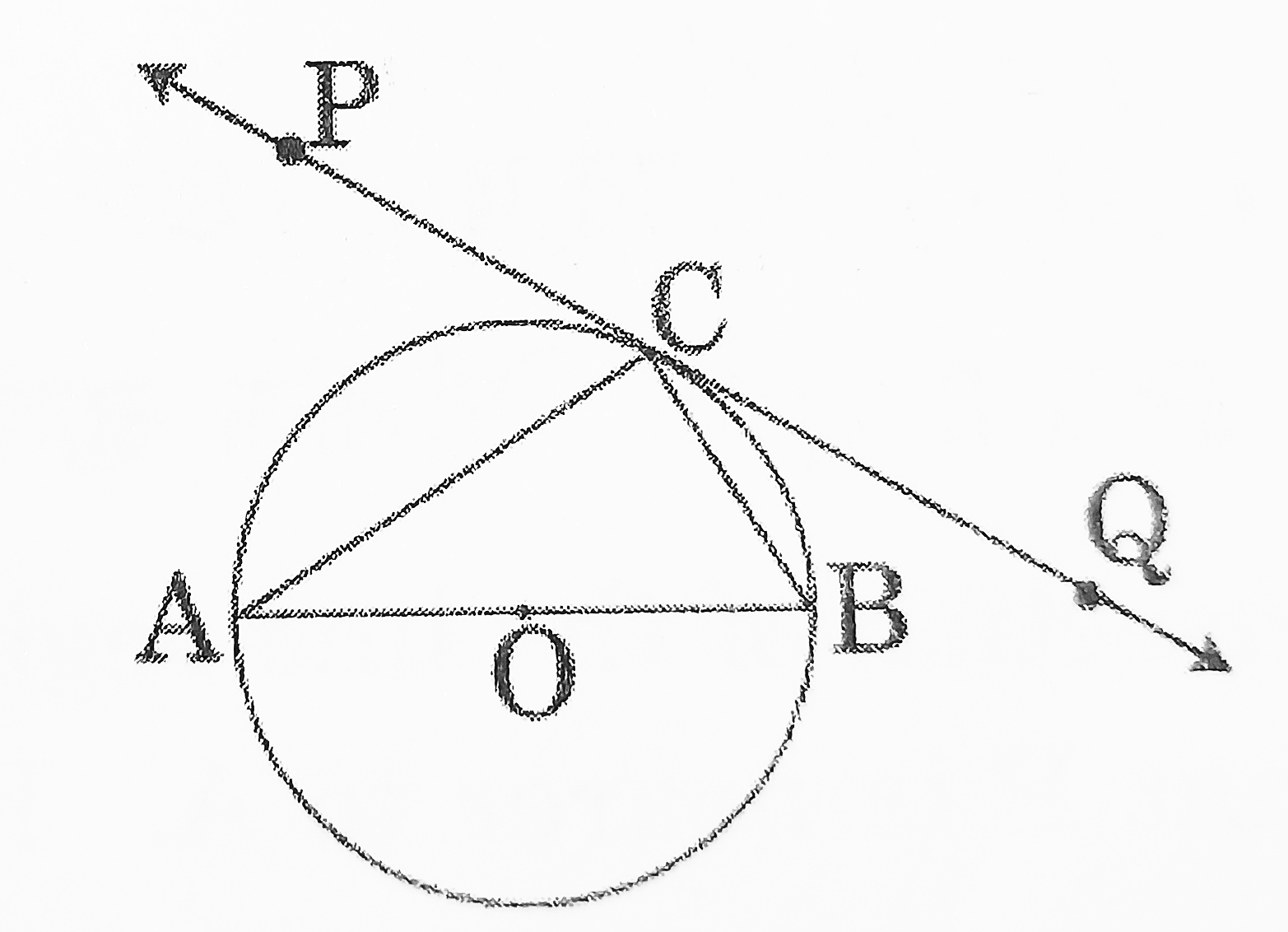[CBSE 2016] [1 Mark]
2. Two concentric circles of radii a and b (a > b) are given. Find the length of the chord of the larger circle which touches the similar circle. [CBSE 2019] [1 Mark]
3. In the given figure, TP and TQ are tangents drawn to the circle with centre at O. If ∠POQ = 115° then ∠PTQ is
1. 115°
2. 57.5°
3. 55°
4. 65°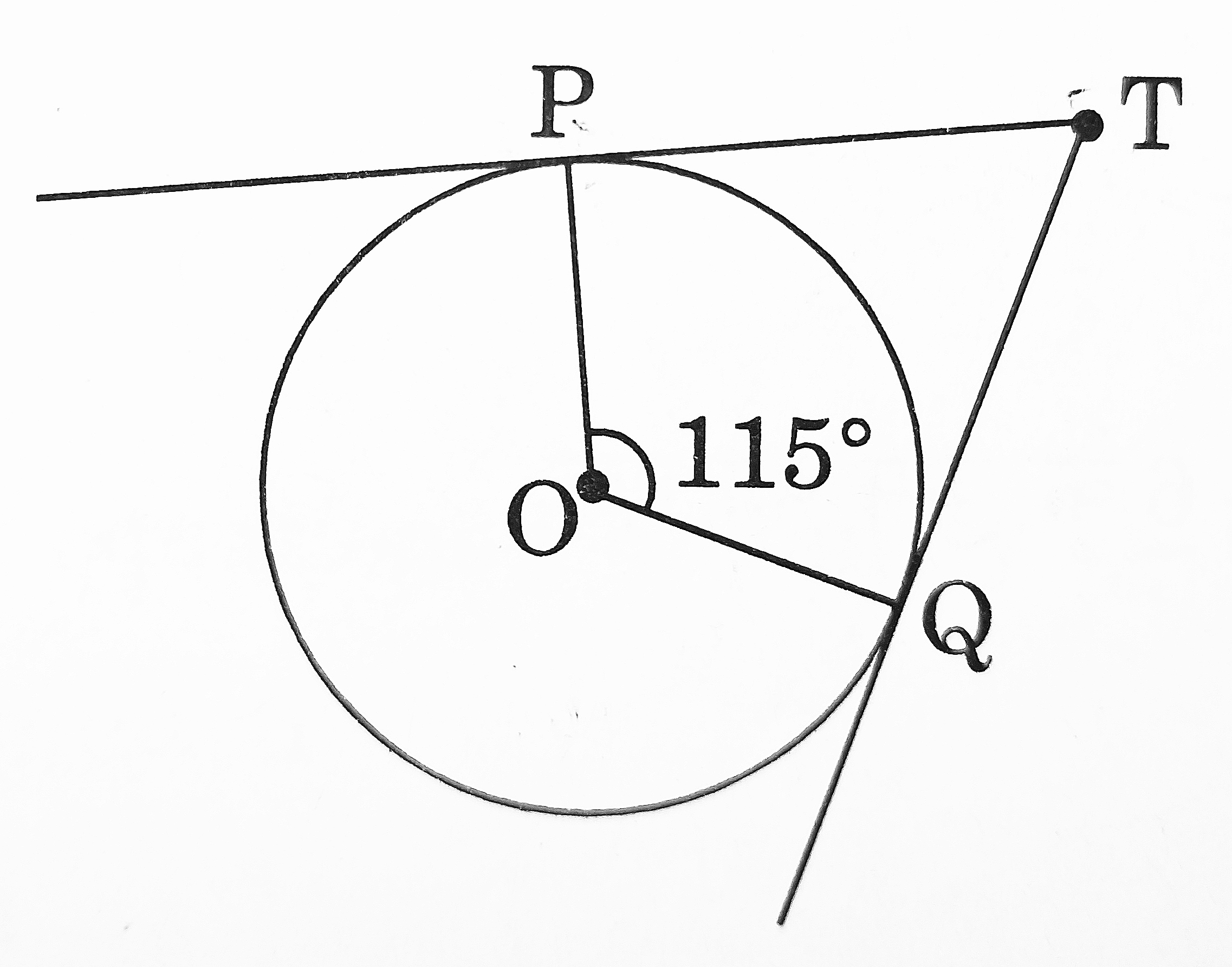[CBSE 2020] [1 Mark]
4. From an external point Q, the length of the tangent to a circle is 5 cm and the distance of Q from the centre is 8 cm. The radius of the circle is
1. 39 cm
2. 3 cm
3. 39 cm
4. 7 cm [CBSE 2020] [1 Mark]
5. In the given figure AB and CD are common tangents to circles which touch each other at D. If AB = 8 cm, then find the length of CD.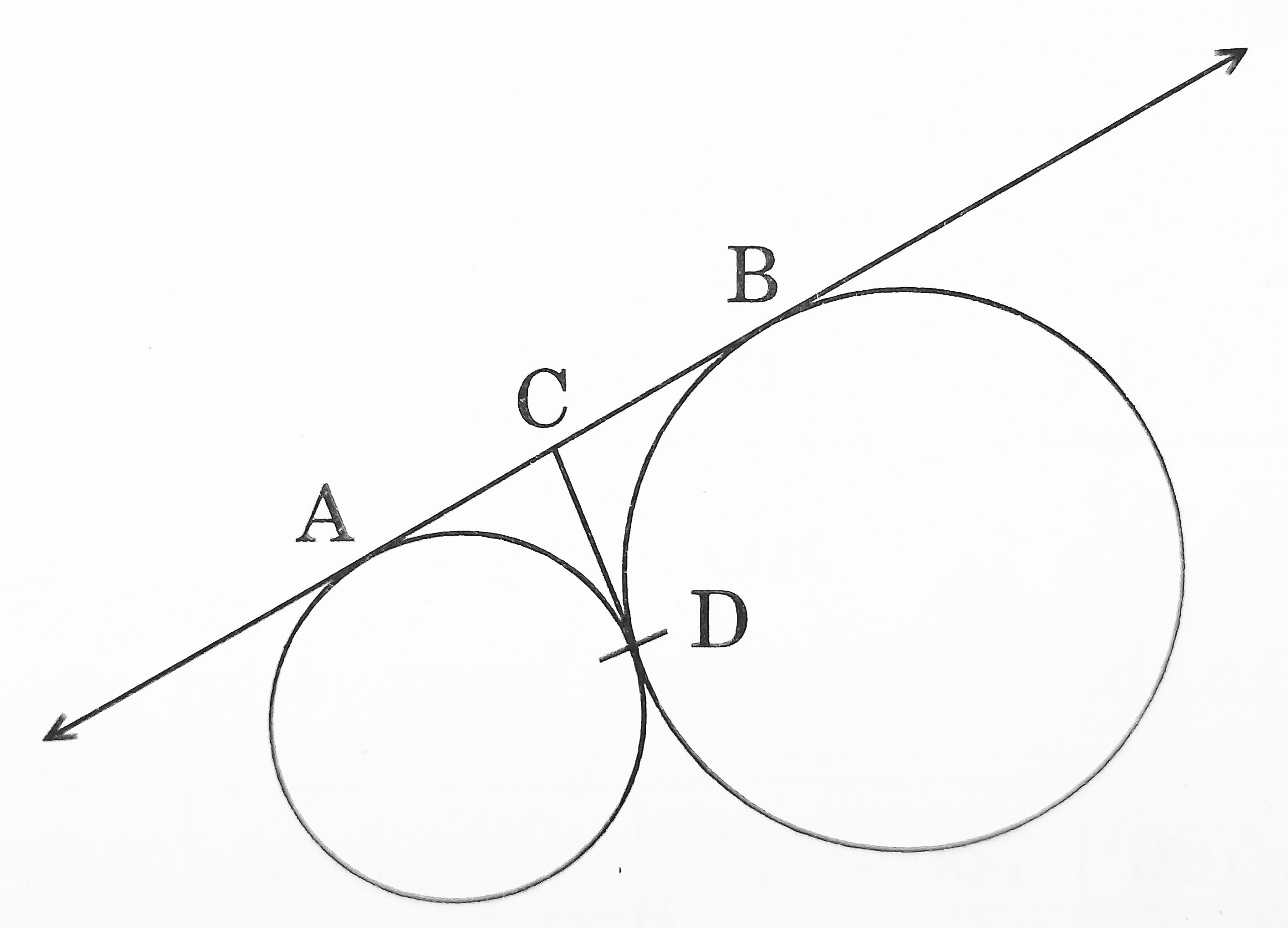[CBSE 2020] [1 Mark]
6. In the given figure a quadrilateral ABCD is drawn to circumscribe a circle, with centre O, in such a way that the sides AB, BC, CD and DA touch the circle at the points P, Q ,R and S respectively. Prove that AB + CD = BC + DA.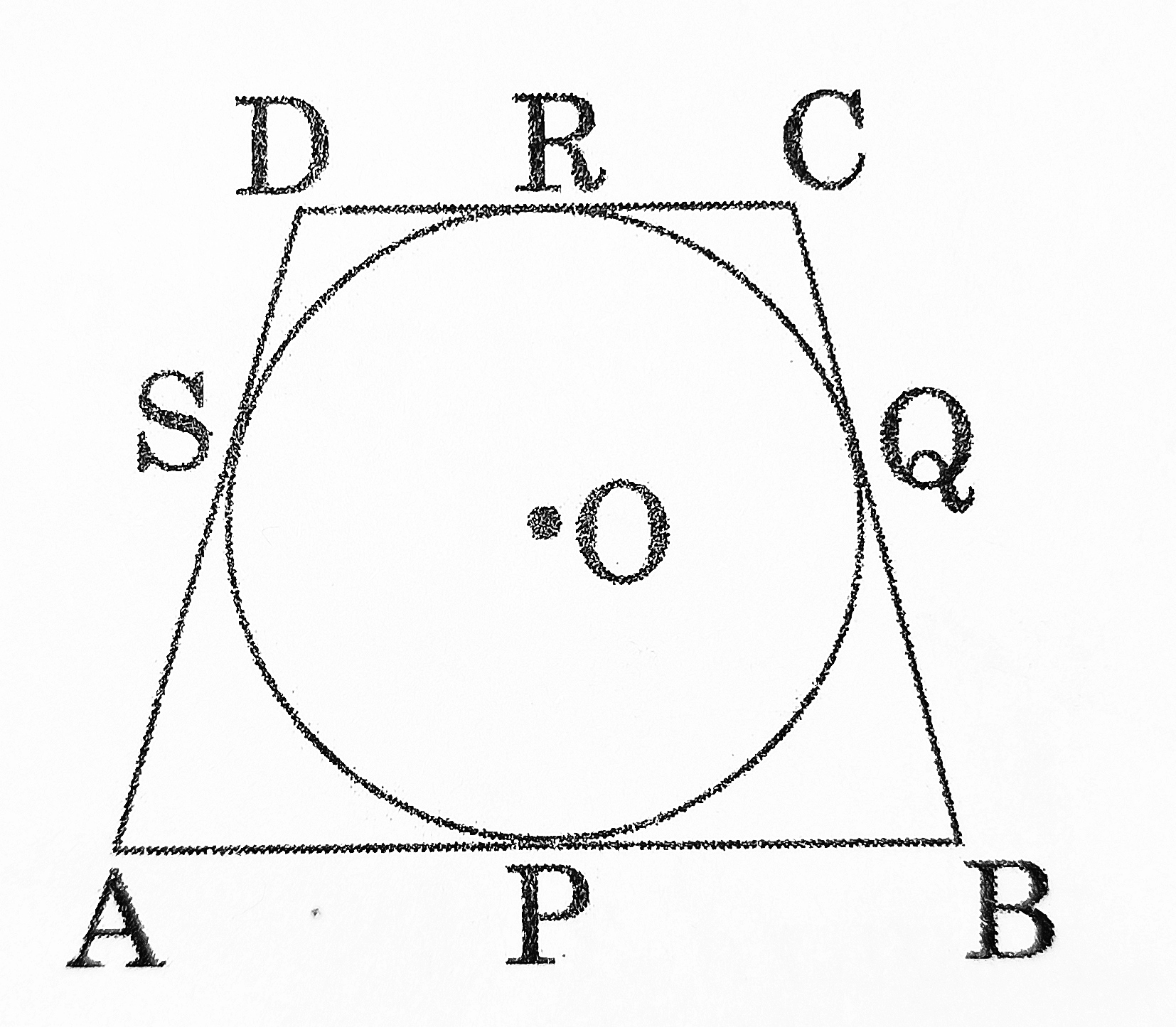[CBSE 2016] [2 Marks]
7. In the given figure, from an external point P, two tangents PT and PS are drawn to a circle with centre O and radius r. If OP = 2r, show that ∠OTS = ∠OST = 30°.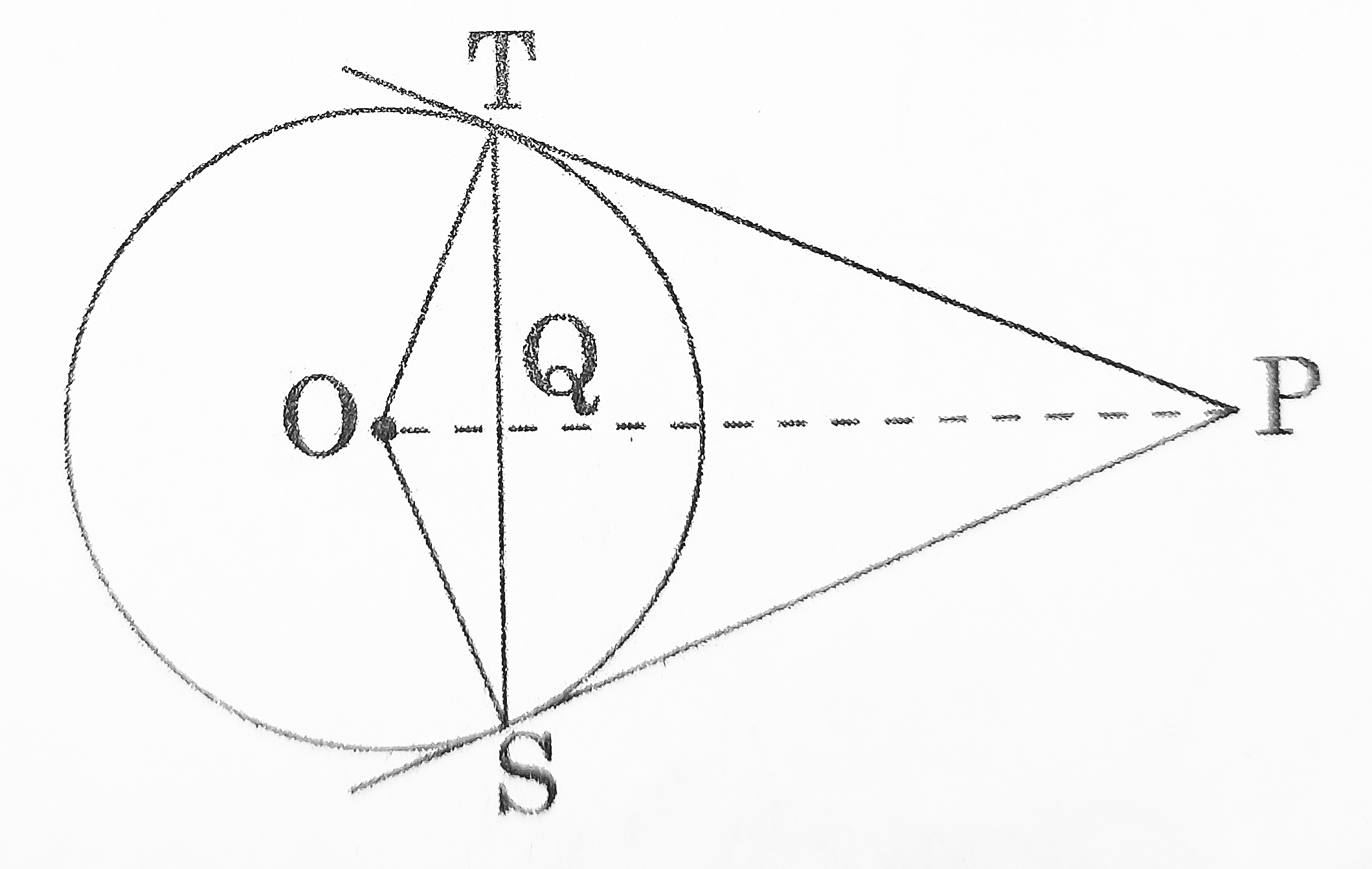[CBSE 2016] [2 Marks]
8. Prove that the lengths of tangents drawn from an external point to a circle are equal. [CBSE 2016] [2 Marks]
9. In the given figure, O is the centre of a circle, PQ is a chord and PT is the tangents at P. If ∠POQ = 70° then find ∠TPQ.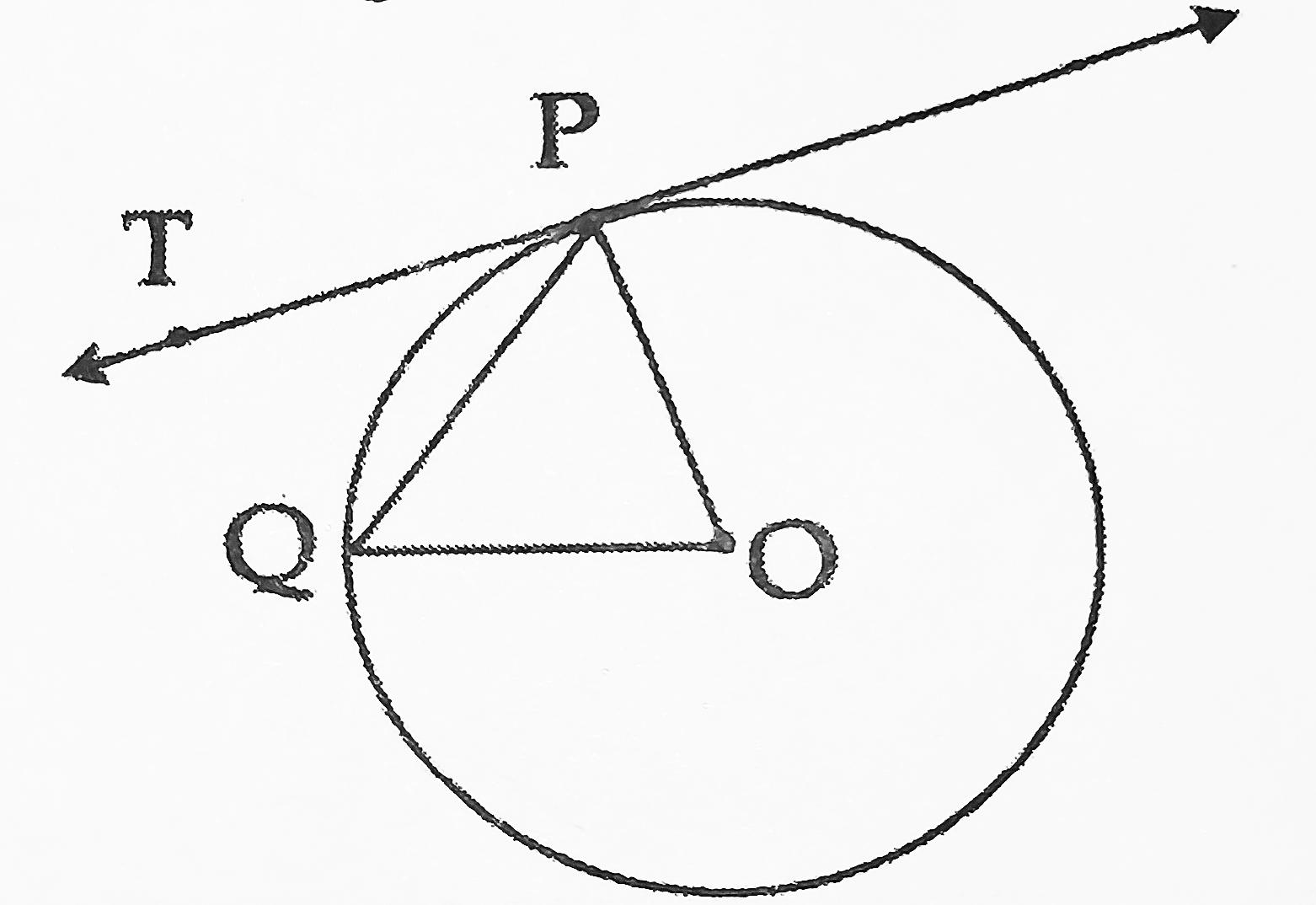[CBSE 2017] [2 Marks]
10. In the given figure, find the length of AP, where O is the centre of circle.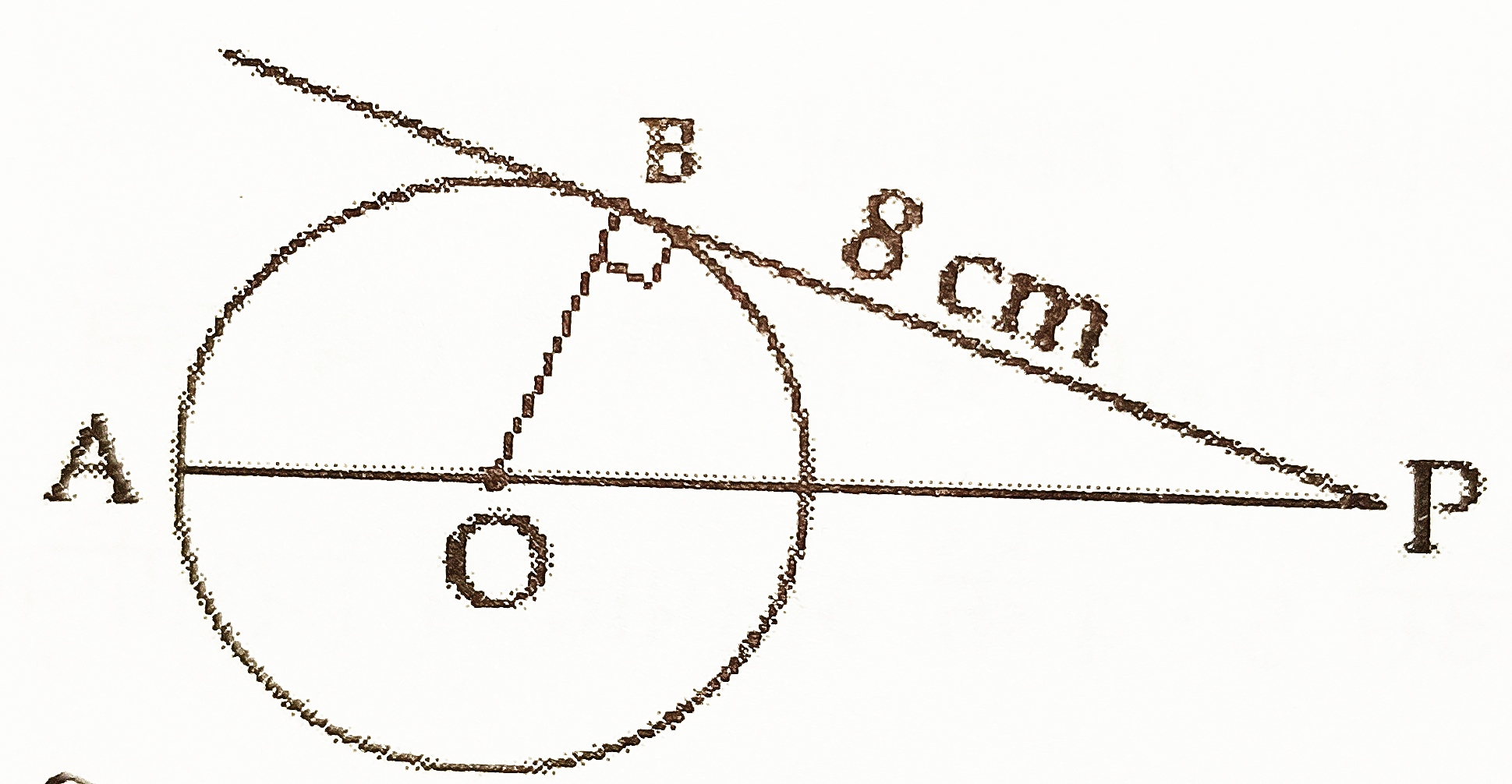[CBSE 2017] [2 Marks]
11. Prove that the lengths of tangents drawn from an external point to a circle are equal. [CBSE 2018] [3 Marks]
12. In the given figure PQ is a chord of length 8 cm of a circle of radius 5 cm. The tangents P and Q intersect at a point T. Find the length TP.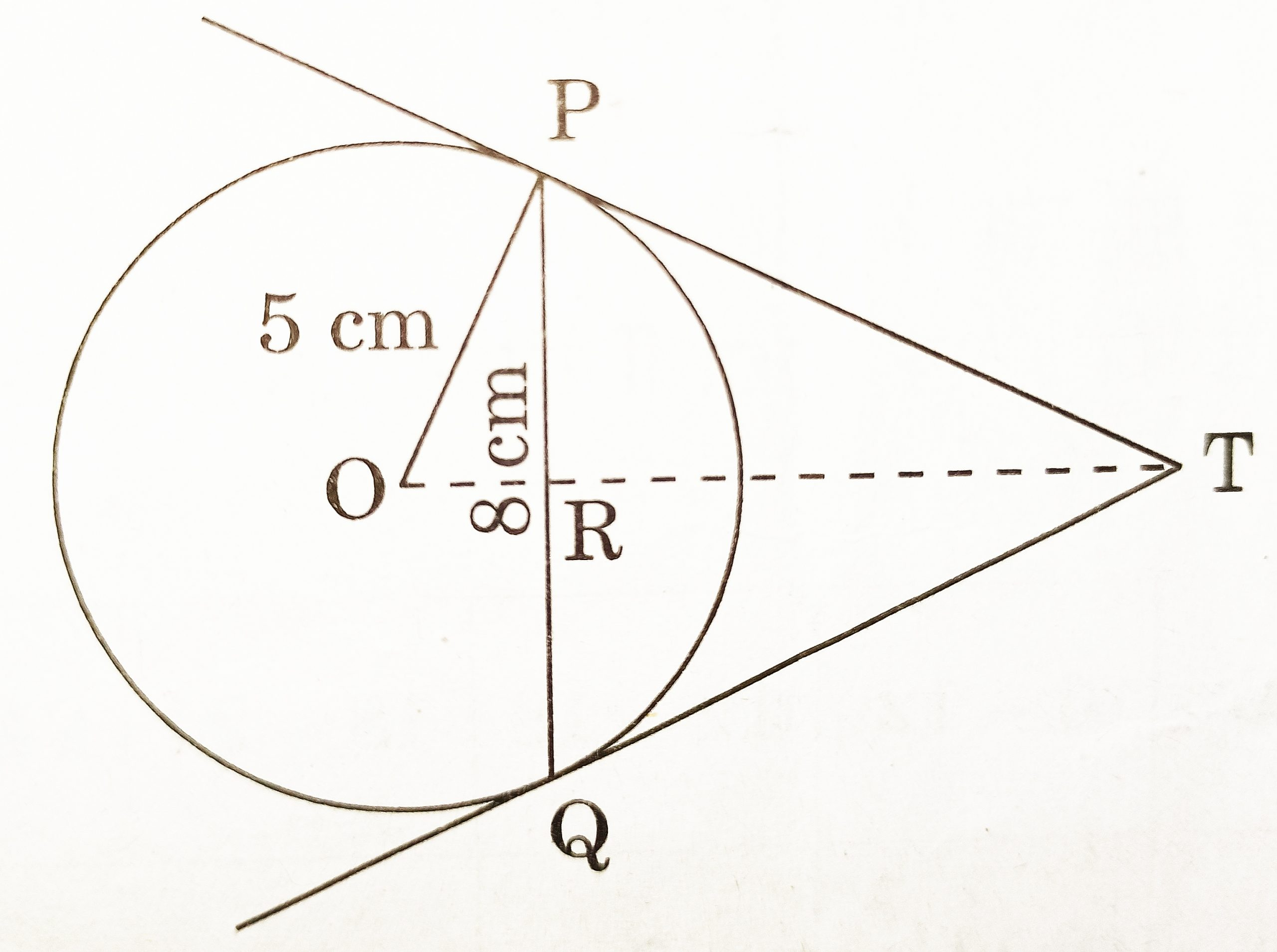[CBSE 2019] [3 Marks]
13. Prove that opposite sides of a quadrilateral circumscribing a circle subtend supplementary angles at the centre of the circle. [CBSE 2019] [3 Marks]
14. In the given figure, XY and MN are two parallel tangents to a circle with centre O and another tangent AB with point of contact C intersecting XY at A and MN at B. Prove that ∠AOB = 90°.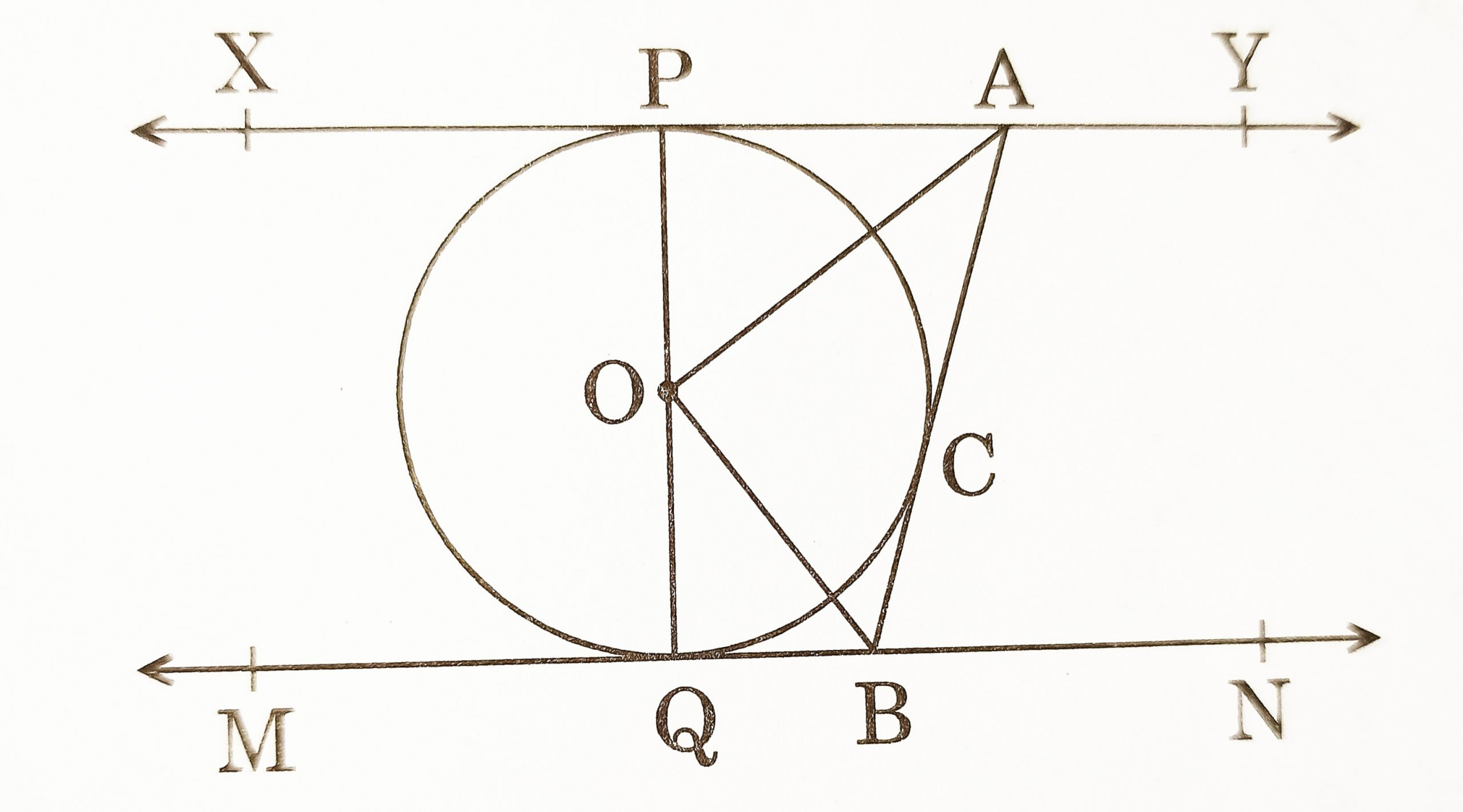[CBSE 2020] [3 Marks]
15. In the given figure, AB is the diameter of a circle with centre O and AC is its chord such that ∠BAC = 30°. If the tangent drawn at C intersects extended AB at D, then show that BC = BD.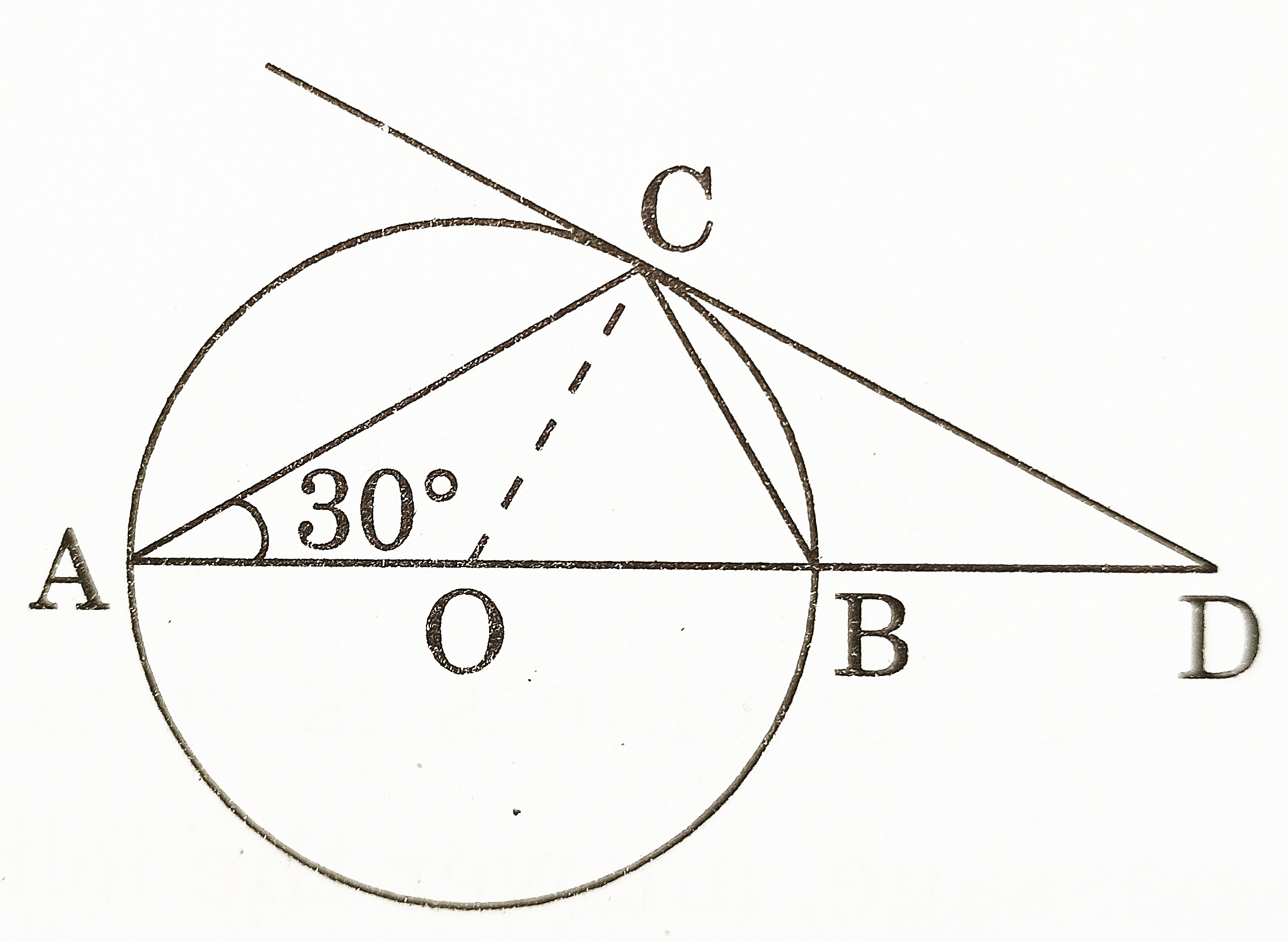[CBSE 2020] [3 Marks]
16. Prove that the lengths of the tangents drawn from an external point to a circle are equal. [CBSE 2016] [4 Marks]
17. In the given figure, two equal circles, with centres O and O, touch each other at X. OO produced meets the circle with centre O’ at A. AC is tangent to circle with centre O, at the point C. OD is perpendicular to AC. Find the value of DO/CO.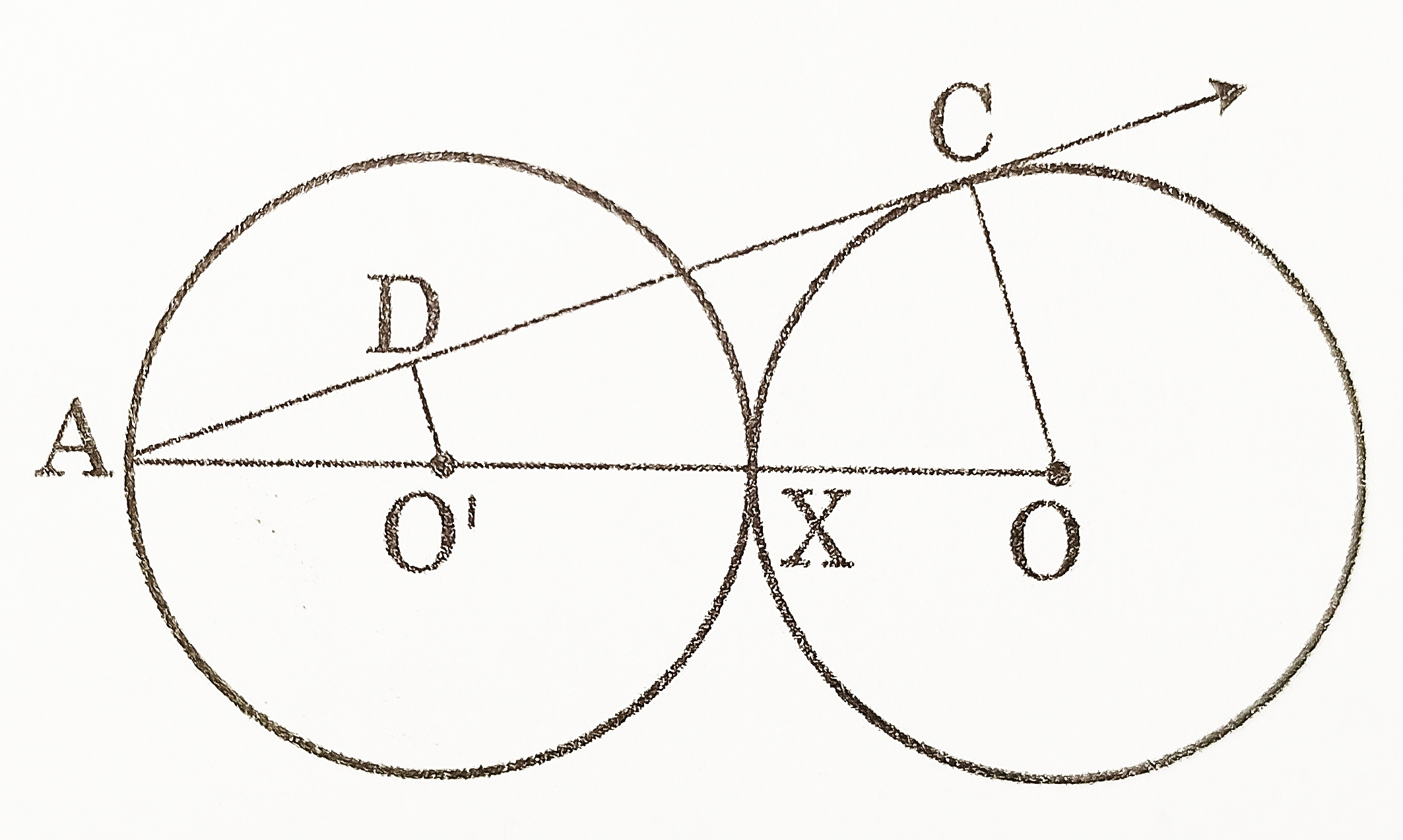[CBSE 2016] [4 Marks]
18. TP and TQ are two tangents drawn to a circle with centre O from an external point T. Prove that ∠PTQ = 2∠OPQ. [CBSE 2017] [4 Marks]
19. A model of a traffic signal on the road has a triangular base ABC with ∠A = 90° and with a red circular light within it as shown in the figure. If AB = 12 cm and BC =20 cm and R is the in centre of the triangle ABC, find the area used for the red light.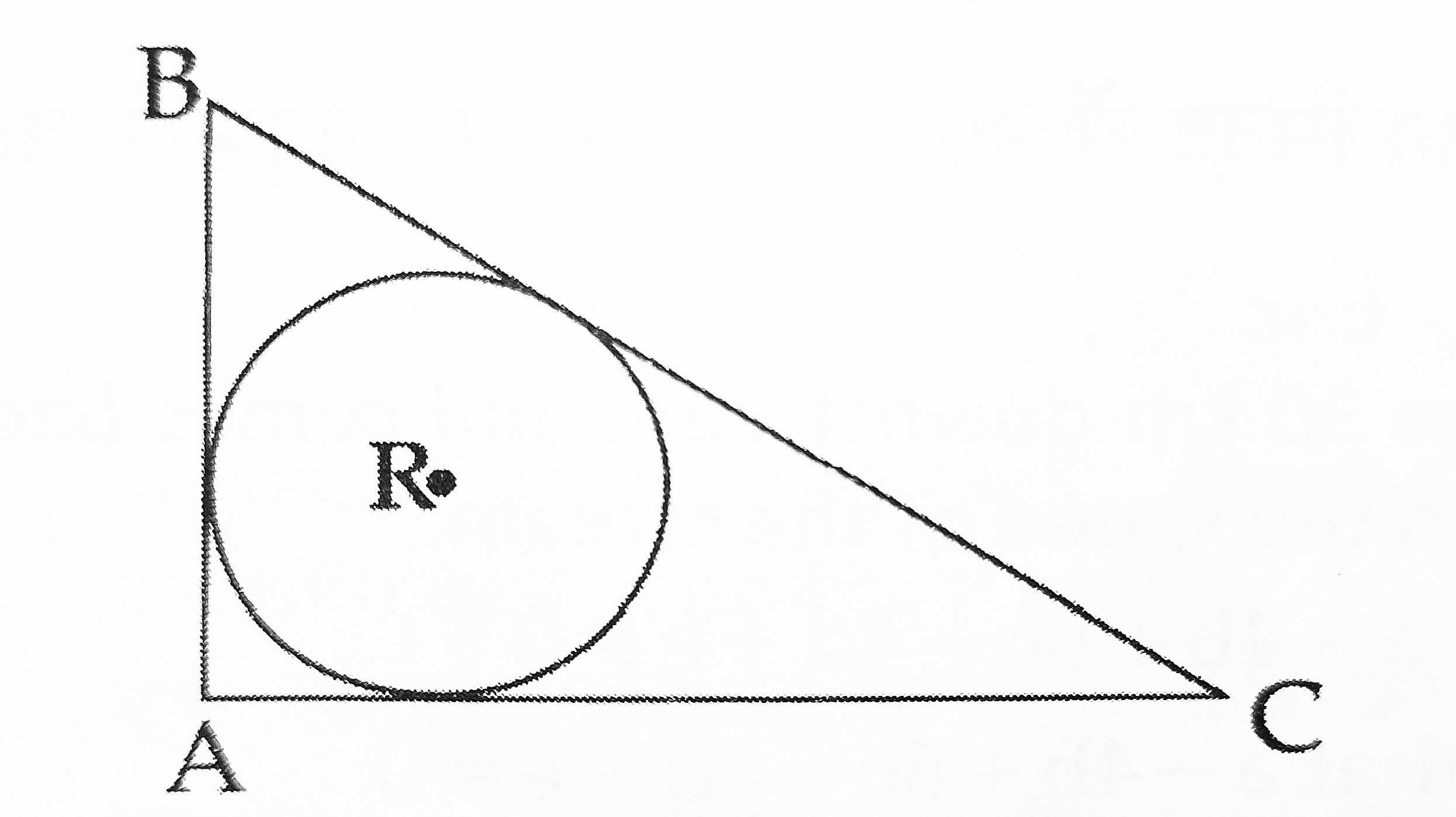[CBSE 2017] [4 Marks]